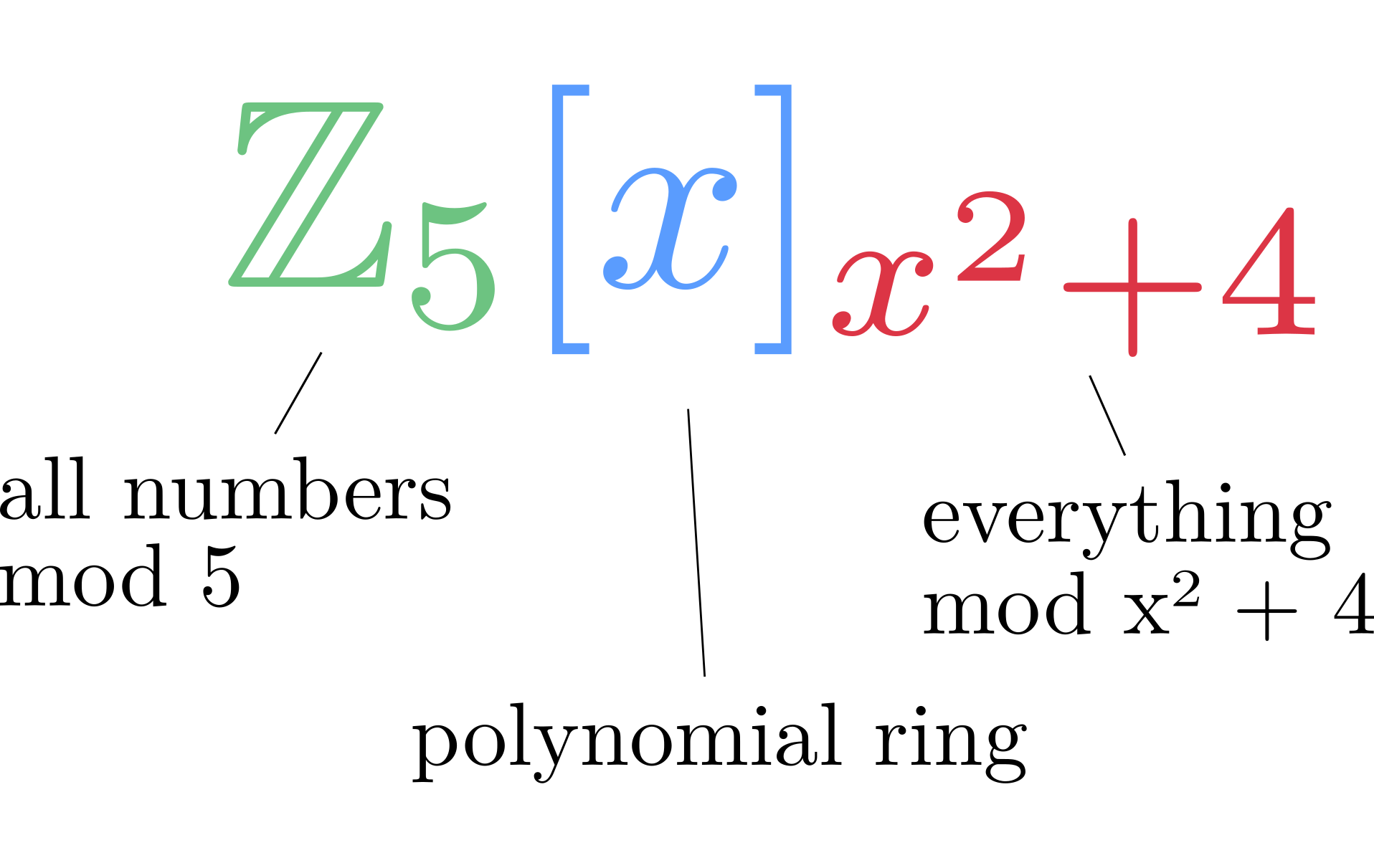Consider the question

Is $A = \mathbb{Z}_{5}[x]_{x^2+4}$ a field? Prove your answer.

What do we do? First, let us try to understand what exactly we have here.So elements of this ring would be $x+3$, $1$ or $4x + 1$. Now, we can use Theorem 5.36, which tells us that the ring $F[x]_{m(x)}$ is a field if and only if $m(x)$ is irreducible. This in general isn’t that easy - if the degree of $m(x)$ is high, this would take quite some manual work (namely using long division to test all possible polynomials with $\le \text{degree}/2$).

Luckily, we are usually asked to show this with $m(x)$ having degree 2 or 3. In this case, we can use Corollary 5.29 (A polynomial $m(x)$ of degree 2 or 3 over a field $F$ is irreducible if and only if it has no root). Hence, we can plug in all possible $a \in F$ into $m(x)$. If $m(a)$ then evaluates to $0$, that means we have found a root, $m(x)$ is not irreducible and $F[x]_{m(x)}$ is not a field. Otherwise, we can conclude that $F[x]_{m(x)}$ indeed is a field.

Let’s now apply this to our initial exercise: We insert all possible values $a \in \mathbb{Z}_5$ into $x^{2} + 4$ and see if this term evaluates to $0$.

$$m(0) = 0^2 + 4 = 4 \newline m(1) = 1^2 + 4 = \textcolor{red}{0} \newline m(2) = 2^2 + 4 = 3 \newline m(3) = 3^2 + 4 = 3 \newline m(4) = 4^2 + 4 = \textcolor{red}{0}$$

(Of course you can also stop as soon as you have found a $0$.)
Clearly, $x^2 + 4$ over $\mathbb{Z}_{5}[x]_{x^2+4}$ does have roots and is therefore not irreducible. We can see that $x^2 + 4 = (x - 1)(x - 4) = (x+4)(x+1)$ by using Lemma 5.28. Finally, we can conclude that $\mathbb{Z}_5[x]_{x^2 + 4}$ is not a field.

For practice, you could now try to prove or disprove that $\mathbb{Z}_5[x]_{x^2 + 2}$ is a field.